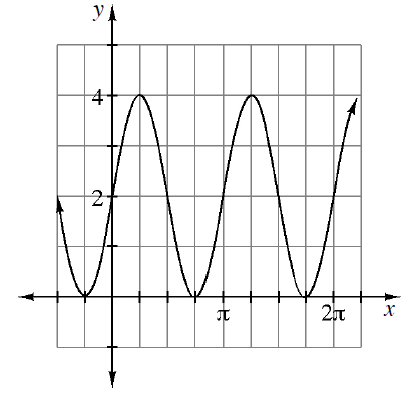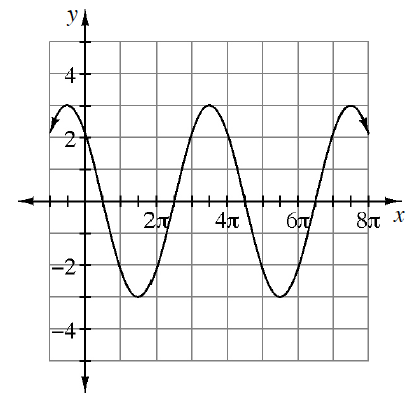### Home > PC3 > Chapter 11 > Lesson 11.1.3 > Problem11-45

11-45.

Write two equations that will generate each of the graphs below, one in terms of sine and one in terms of cosine.

1.Using a sine function, the amplitude is $2$, the period is π, there is no horizontal shift, and the vertical shift is $2$.

1.Using a sine function, it is vertically reflected with an amplitude of $3$, a period of $4π$, a horizontal shift of $π/2$, and no vertical shift.